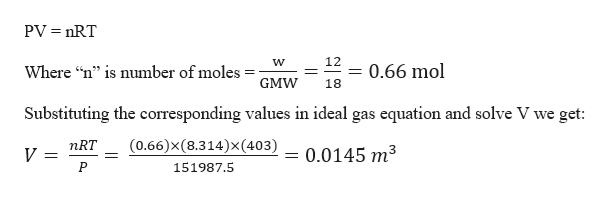# Balloons need to contain gas lighter than air to float. Water is lighter than air when vaporized (18 g/mol vs. 28.9 g/mol) A) 12 g of water are vaporized and raised to 130C, then contained in a perfectly insulated balloon which holds it at a pressure of 1.5 atm. What volume does the balloon take up once inflated in this manner? B) The balloon is then placed in a vacuum chamber containing only 12 g of ice at -10 C. The balloon is popped and the steam intermingles with the ice. What remains in the chamber after the system has come to thermal equilibrium?L fusion = 333 kJ/kg, L vap = 2260 kJ/kg, c steam = 2090 J/kg*C,c water = 4186 J/kg* C, c ice = 2010 J/kg* C

Question
71 views
Balloons need to contain gas lighter than air to float. Water is lighter than air when vaporized (18 g/mol vs. 28.9 g/mol)

A) 12 g of water are vaporized and raised to 130C, then contained in a perfectly insulated balloon which holds it at a pressure of 1.5 atm. What volume does the balloon take up once inflated in this manner?

B) The balloon is then placed in a vacuum chamber containing only 12 g of ice at -10 C. The balloon is popped and the steam intermingles with the ice. What remains in the chamber after the system has come to thermal equilibrium?
L fusion = 333 kJ/kg, L vap = 2260 kJ/kg, c steam = 2090 J/kg*C,
c water = 4186 J/kg* C, c ice = 2010 J/kg* C
check_circle

Step 1

Given information:

Gram molecular weight of water (GMW) = 18 g

Weight of vapour filled in the balloon (w) = 12 g

Pressure at which it is filled (P) = 1.5 atm

Temperature at which the vapour exists (t) = 130 0C

Step 2

Volume occupied by the vapour at the given conditions be (V) =?

We know that a pressure of 1 atm = 101325 N/m2

Then the pressure 1.5 atm = 151987.5 N/m2

Universal gas constant (R) = 8.314 J/mol. K

Given temperature in kelvin scale is (T) = t + 273 0K = 130 + 273 = 403 0K

We know that the ideal gas equation is:help_outlineImage TranscriptionclosePV nRT 12 0.66 mol Where "n" is number of moles _ 11 GMW 18 Substituting the corresponding values in ideal gas equation and solve V we get: (0.66)x(8.314)x(403) nRT 0.0145 m3 V P 151987.5 fullscreen
Step 3

Part B:

Given information:

Mass of ice (M) = 12 g = 0.012 kg

Mass of steam (m) = 12 g = 0.012 kg

Temperature of ice (Tice) = -10 0C

Temperature of water (Tsteam) = 130 0C

L fusion = 333 kJ/kg, L vapour = 2260 kJ/kg,

Csteam = 2090 J/kg...

### Want to see the full answer?

See Solution

#### Want to see this answer and more?

Solutions are written by subject experts who are available 24/7. Questions are typically answered within 1 hour.*

See Solution
*Response times may vary by subject and question.
Tagged in

### Thermodynamics